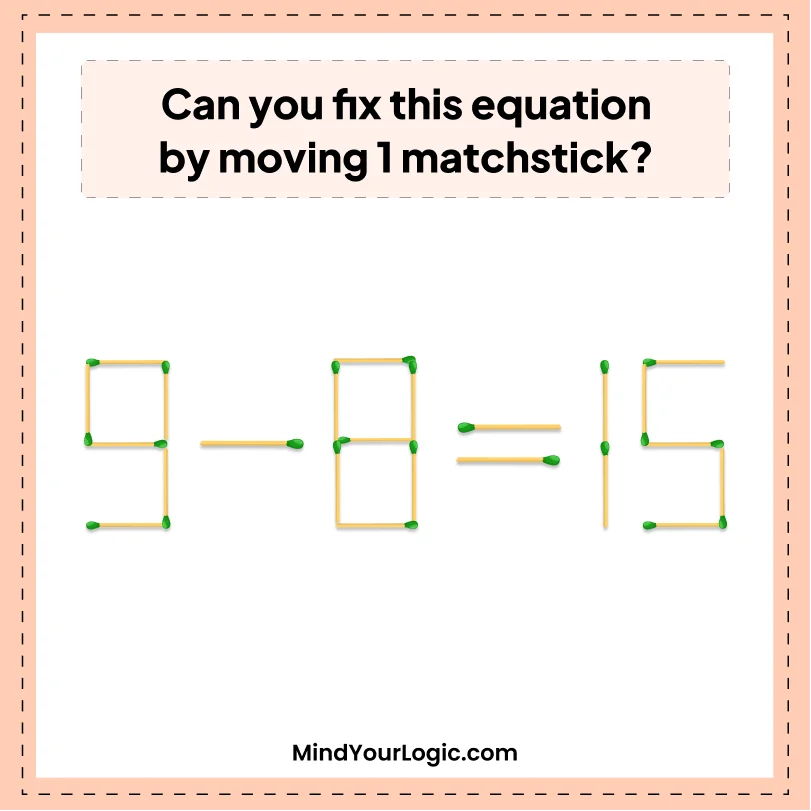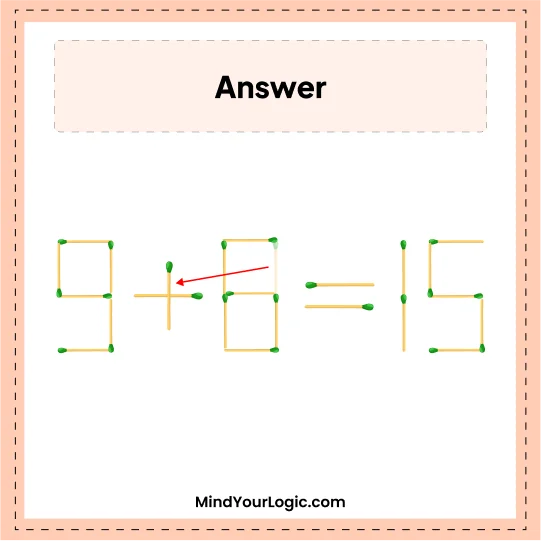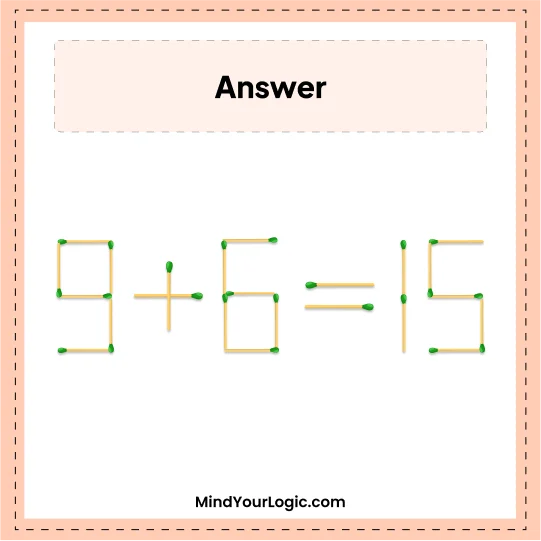# Move 1 and Correct 9-8=15 - Matchstick Puzzle

###### 35.Matchstick Puzzles
```Can you fix this equation by moving just 1 matchstick?
```•

Explanation :

` By removing the top left matchstick change the 8 into a 6 and use that matchstick to change the minus into a plus. So, get correct equation 9 + 6 = 15.`